Who We Are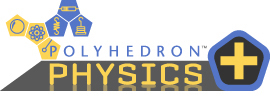Polyhedron Physics+ is a remarkably realistic collection of simulated physics laboratory equipment and data collection tools. 24 iOS iPad apps for high school level physics have everything you need to conduct physics laboratory experiments. 3D simulations that produce realistic data, PLUS introductory videos, background theory, experimental procedures, and analysis and reporting tools. Teacher support materials include Post-lab Quizzes, Questions with sample answers, and sample Lab Reports. Polyhedron Physics+ is an ideal option when hands-on lab equipment is not available, and when remote learning is desirable or essential.

TEACHER SUPPORT MATERIALS

E-mail Tech Support for
Polyhedron Physics Apps

See Our Work
Send Us Email PROJECTS:
Home • Current
• Completed
• iOS Apps

Polyhedron Physics+ Apps

iNSIGHT Apps

Other Apps

 Polyhedron Physics+ Apps are now available as web-based simulations! To learn more, visit PolyhedronPhysics.com for product details and purchasing information.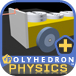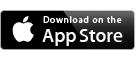NEWTON'S SECOND
LAW OF MOTION

Use a laboratory cart to investigate the relationships between the force applied to an object and the mass and acceleration of the object.ACCELERATION OF
GRAVITY

Use a position sensor to examine the relationship between the position, velocity, and acceleration of a free-falling body.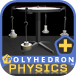FORCE VECTORS

Uses a force table to investigate the vector addition of forces.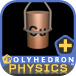ARCHIMEDES’ PRINCIPLE

Use a laboratory balance, metal samples, water, and alcohol to investigate Archimedes’ Principle, and to determine the mass densities of solids and fluids.CENTRIPETAL FORCE

Use a centripetal force apparatus to investigate the relationships between the centripetal force on an object and the mass, velocity, and radius of revolution of the object.CONSERVATION
OF ENERGY

Use a spring-mass system to investigate the conservation of mechanical energy.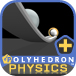CONSERVATION OF MOMENTUM
Use two colliding bodies to investigate elastic and inelastic collisions, and compare kinetic energy.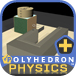FRICTION

Use a friction block to determine the coefficients of static friction and kinetic friction for two surfaces in contact.GAS LAWS

Use a syringe, masses, a thermometer, a hotplate, and a water and ice bath to investigate Boyle’s Law and Charles’s Law.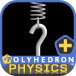HOOKE'S LAW

Use a spring-mass system to investigate the relationship between the force acting on a spring and the resulting change in length of the spring.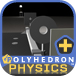IMAGE FORMATION
USING LENSES

Use an optical bench to determine the focal length of a converging lens and to investigate the relationship between the object distance and the image distance.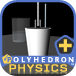JOULE HEATING

Use a calorimeter to investigate electrical energy and thermal energy.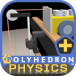MOMENTUM
AND IMPULSE

Uses a laboratory cart to investigate the relationship between the impulse exerted on a constant mass and the resulting change in the momentum of the mass.MOTION OF
A PENDULUM

Use a mass on a string to investigate the relationships between the mass, length, amplitude, and period of oscillation of a pendulum.OHM’S LAW

Use a resistor, a power supply, a voltmeter, and an ammeter to investigate the relationships between the voltage, current, and resistance in a circuit.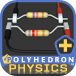RC CIRCUITS

Use a power supply, voltmeters, capacitors, and resistors to investigate RC circuits.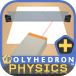REFLECTION
OF LIGHT

Use a plane mirror to investigate the relationships between object and image distances and angles of incidence and reflection.REFRACTION
OF LIGHT

Use a refraction cell and a ray box to investigate Snell’s Law of Refraction, total internal reflection, and the critical angle of refraction.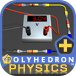SERIES AND
PARALLEL CIRCUITS

Use resistors, a power supply, a voltmeter, and an ammeter to investigate the effective resistances of circuits with resistors in series, in parallel, and in combinations of series and parallel components.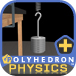SIMPLE HARMONIC MOTION

Use a spring-mass system to investigate relationships between the spring constant, mass, amplitude of oscillation, and period of oscillation for a mass on a spring.SPECIFIC HEAT
OF A METAL

Use a calorimeter to measure the specific heat of a metal.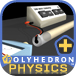SPEED OF SOUND

Use a resonance chamber to measure the wavelength of a sound wave, and determine an experimental value for the speed of sound in air.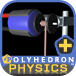WORK AND ENERGY USING AN ELECTRIC MOTOR

Use an electric motor and other equipment to investigate energy efficiency.WORK AND MECHANICAL ENERGY

Use a laboratory cart to investigate the relationship between the work done on an object and the change in kinetic energy of the object.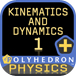KINEMATICS AND DYNAMICS — BUNDLE 1 Explore the physics of forces, gravity, acceleration, work, and energy. Acceleration of Gravity Addition of Force Vectors Work and Energy Using an Electric Motor Work and Mechanical EnergyKINEMATICS AND DYNAMICS — BUNDLE 2 Explore the physics of centripetal force, momentum, impulse, collisions, and friction. Centripetal Force Conservation of Momentum Friction Momentum and ImpulseKINEMATICS AND DYNAMICS — BUNDLE 3 Explore the physics of springs, spring-mass systems, simple harmonic motion, conservation of mechanical energy, and the simple pendulum. Conservation of Energy Hooke’s Law Motion of a Pendulum Simple Harmonic Motion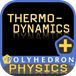THERMODYNAMICS BUNDLE Explore the physics of density, buoyancy, pressure, temperature, and heat. Archimedes’ Principle Gas Laws Joule Heating Specific Heat of a Metal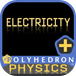ELECTRICITY BUNDLE Explore the physics of electrical circuits, resistance, capacitance, work, and energy. Ohm’s Law RC Circuits Series and Parallel Circuits Work and Energy Using an Electric Motor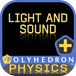LIGHT AND SOUND BUNDLE Explore the physics of reflection, refraction, lenses, resonance, and propagation of waves. Image Formation Using Lenses Reflection of Light Refraction of Light Speed of Sound——